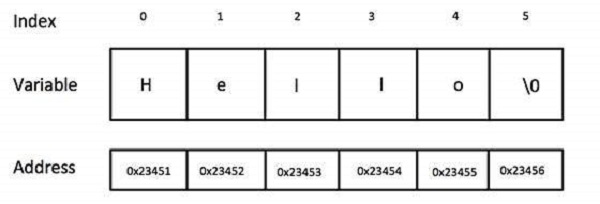# Computer Programming - Strings

During our discussion about characters, we learnt that character data type deals with a single character and you can assign any character from your keyboard to a character type variable.

Now, let's move a little bit ahead and consider a situation where we need to store more than one character in a variable. We have seen that C programming does not allow to store more than one character in a character type variable. So the following statements are invalid in C programming and produce syntax errors −

```char ch1 = 'ab';
char ch2 = '10';
```

We have also seen how to use the concept of arrays to store more than one value of similar data type in a variable. Here is the syntax to store and print five numbers in an array of int type −

```#include <stdio.h>

main() {
int number = {10, 20, 30, 40, 50};
int i = 0;

while( i < 5 ) {
printf("number[%d] = %d\n", i, number[i] );
i = i + 1;
}
}
```

When the above code is compiled and executed, it produces the following result −

```number = 10
number = 20
number = 30
number = 40
number = 50
```

Now, let's define an array of five characters in the same way as we did for numbers and try to print them −

```#include <stdio.h>

main() {
char ch = {'H', 'e', 'l', 'l', 'o'};
int i = 0;

while( i < 5 ) {
printf("ch[%d] = %c\n", i, ch[i] );
i = i + 1;
}
}
```

Here, we used %c to print character value. When the above code is compiled and executed, it produces the following result −

```ch = H
ch = e
ch = l
ch = l
ch = o
```

If you are done with the above example, then I think you understood how strings work in C programming, because strings in C are represented as arrays of characters. C programming simplified the assignment and printing of strings. Let's check the same example once again with a simplified syntax −

```#include <stdio.h>

main() {
char ch = "Hello";
int i = 0;

/* Print as a complete string */
printf("String = %s\n", ch);

/* Print character by character */
while( i < 5 ) {
printf("ch[%d] = %c\n", i, ch[i] );
i = i + 1;
}
}
```

Here, we used %s to print the full string value using array name ch, which is actually the beginning of the memory address holding ch variable as shown below −Although it's not visible from the above examples, a C program internally assigns null character '\0' as the last character of every string. It indicates the end of the string and it means if you want to store a 5 character string in an array, then you must define an array size of 6 as a good practice, though C does not complain about it.

If the above code is compiled and executed, it produces the following result −

```String = Hello
ch = H
ch = e
ch = l
ch = l
ch = o
```

## Basic String Concepts

Based on the above discussion, we can conclude the following important points about strings in C programming language −

• Strings in C are represented as arrays of characters.

• We can constitute a string in C programming by assigning character by character into an array of characters.

• We can constitute a string in C programming by assigning a complete string enclosed in double quote.

• We can print a string character by character using an array subscript or a complete string by using an array name without subscript.

• The last character of every string is a null character, i.e., ‘\0’.

• Most of the programming languages provide built-in functions to manipulate strings, i.e., you can concatenate strings, you can search from a string, you can extract sub-strings from a string, etc. For more, you can check our detailed tutorial on C programming or any other programming language.

## Strings in Java

Though you can use character arrays to store strings, but Java is an advanced programming language and its designers tried to provide additional functionality. Java provides strings as a built-in data type like any other data type. It means you can define strings directly instead of defining them as array of characters.

Following is the equivalent program written in Java. Java makes use of the new operator to create string variables as shown in the following program.

You can try to execute the following program to see the output −

```public class DemoJava {
public static void main(String []args) {
String str = new String("Hello");
System.out.println( "String = " + str );
}
}
```

When the above program is executed, it produces the following result −

```String = Hello
```

## Strings in Python

Creating strings in Python is as simple as assigning a string into a Python variable using single or double quotes.

Given below is a simple program that creates two strings and prints them using print() function −

```var1 = 'Hello World!'
var2 = "Python Programming"

print "var1 = ", var1
print "var2 = ", var2
```

When the above program is executed, it produces the following result −

```var1 =  Hello World!
var2 =  Python Programming
```

Python does not support character type; these are treated as strings of length one, thus also considered a substring.

To access substrings, use the square brackets for slicing along with the index or indices to obtain your substring. Take a look at the following code segment −

```var1 = 'Hello World!'
var2 = "Python Programming"

print "var1: ", var1
print "var2[1:5]: ", var2[1:5]
```

When the above code is executed, it produces the following result −

```var1:  H
var2[1:5]:  ytho
```